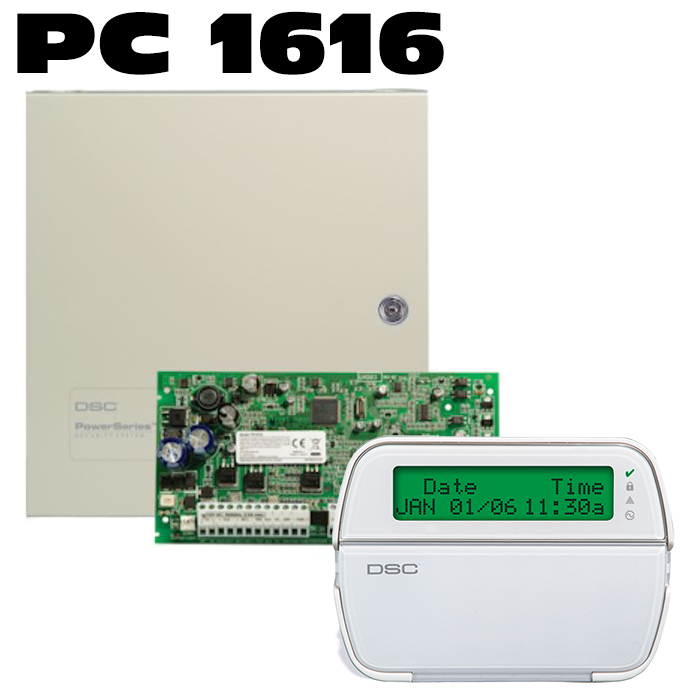To set the DSC Powerseries Pc1616 system time and date you will need to know the master code for the System. You will need to follow these steps " Enter *6 + 4-digit Master User Code + 1 + Time in Military Format + 2 digit month + 2 digit day + 2 Digit Year + # "

Military time for the DSC PC1616 system  is as follows :

00:00 = 12am
01:00 = 1am
02:00 = 2am
03:00 = 3am
04:00 = 4am
05:00 = 5am
06:00 = 6am
07:00 = 7am
08:00 = 8am
09:00 = 9am
10:00 = 10am
11:00 = 11am
12:00 = 12pm
13:00 = 1pm
14:00 = 2pm
15:00 = 3pm
16:00 = 4pm
17:00 = 5pm
18:00 = 6pm
19:00 = 7pm
20:00 = 8pm
21:00 = 9pm
22:00 = 10pm
23:00 = 11pm

DSC Pc1616 Alarm Monitoring Options

DSC Pc1616 Frequently Asked Questions